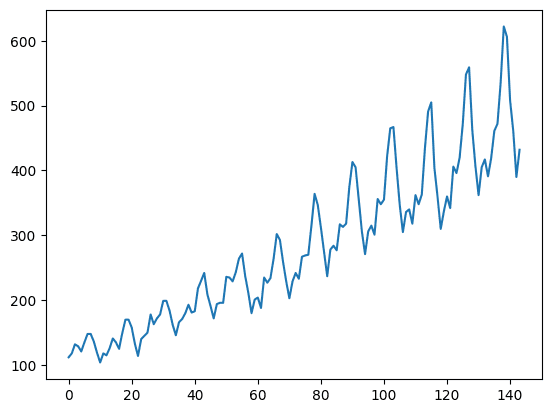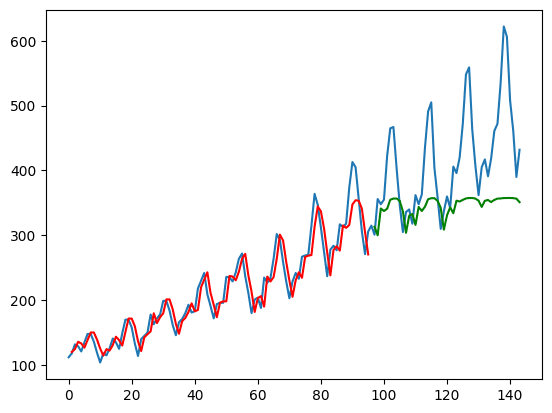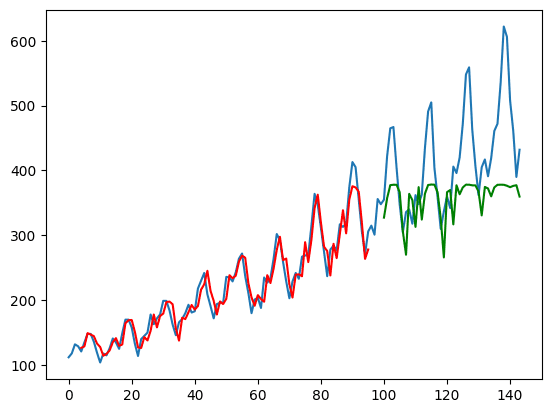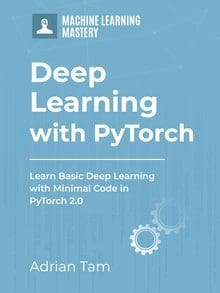# LSTM for Time Series Prediction in PyTorch

Last Updated on April 8, 2023

Long Short-Term Memory (LSTM) is a structure that can be used in neural network. It is a type of recurrent neural network (RNN) that expects the input in the form of a sequence of features. It is useful for data such as time series or string of text. In this post, you will learn about LSTM networks. In particular,

• What is LSTM and how they are different
• How to develop LSTM network for time series prediction
• How to train a LSTM network

Kick-start your project with my book Deep Learning with PyTorch. It provides self-study tutorials with working code.

Let’s get started.LSTM for Time Series Prediction in PyTorch
Photo by Carry Kung. Some rights reserved.

## Overview

This post is divided into three parts; they are

• Overview of LSTM Network
• LSTM for Time Series Prediction
• Training and Verifying Your LSTM Network

## Overview of LSTM Network

LSTM cell is a building block that you can use to build a larger neural network. While the common building block such as fully-connected layer are merely matrix multiplication of the weight tensor and the input to produce an output tensor, LSTM module is much more complex.

A typical LSTM cell is illustrated as followsLSTM cell. Illustration from Wikipedia.

It takes one time step of an input tensor $x$ as well as a cell memory $c$ and a hidden state $h$. The cell memory and hidden state can be initialized to zero at the beginning. Then within the LSTM cell, $x$, $c$, and $h$ will be multiplied by separate weight tensors and pass through some activation functions a few times. The result is the updated cell memory and hidden state. These updated $c$ and $h$ will be used on the **next time step** of the input tensor. Until the end of the last time step, the output of the LSTM cell will be its cell memory and hidden state.

Specifically, the equation of one LSTM cell is as follows:

\begin{aligned} f_t &= \sigma_g(W_{f} x_t + U_{f} h_{t-1} + b_f) \\ i_t &= \sigma_g(W_{i} x_t + U_{i} h_{t-1} + b_i) \\ o_t &= \sigma_g(W_{o} x_t + U_{o} h_{t-1} + b_o) \\ \tilde{c}_t &= \sigma_c(W_{c} x_t + U_{c} h_{t-1} + b_c) \\ c_t &= f_t \odot c_{t-1} + i_t \odot \tilde{c}_t \\ h_t &= o_t \odot \sigma_h(c_t) \end{aligned}

Where $W$, $U$, $b$ are trainable parameters of the LSTM cell. Each equation above is computed for each time step, hence with subscript $t$. These trainable parameters are reused for all the time steps. This nature of shared parameter bring the memory power to the LSTM.

Note that the above is only one design of the LSTM. There are multiple variations in the literature.

Since the LSTM cell expects the input $x$ in the form of multiple time steps, each input sample should be a 2D tensors: One dimension for time and another dimension for features. The power of an LSTM cell depends on the size of the hidden state or cell memory, which usually has a larger dimension than the number of features in the input.

### Want to Get Started With Deep Learning with PyTorch?

Take my free email crash course now (with sample code).

Click to sign-up and also get a free PDF Ebook version of the course.

## LSTM for Time Series Prediction

Let’s see how LSTM can be used to build a time series prediction neural network with an example.

The problem you will look at in this post is the international airline passengers prediction problem. This is a problem where, given a year and a month, the task is to predict the number of international airline passengers in units of 1,000. The data ranges from January 1949 to December 1960, or 12 years, with 144 observations.

It is a regression problem. That is, given the number of passengers (in unit of 1,000) the recent months, what is the number of passengers the next month. The dataset has only one feature: The number of passengers.

Save this file as airline-passengers.csv in the local directory for the following.

Below is a sample of the first few lines of the file:

The data has two columns, the month and the number of passengers. Since the data are arranged in chronological order, you can take only the number of passenger to make a single-feature time series. Below you will use pandas library to read the CSV file and convert it into a 2D numpy array, then plot it using matplotlib:This time series has 144 time steps. You can see from the plot that there is an upward trend. There are also some periodicity in the dataset that corresponds to the summer holiday period in the northern hemisphere. Usually a time series should be “detrended” to remove the linear trend component and normalized before processing. For simplicity, these are skipped in this project.

To demonstrate the predictive power of our model, the time series is splitted into training and test sets. Unlike other dataset, usually time series data are splitted without shuffling. That is, the training set is the first half of time series and the remaining will be used as the test set. This can be easily done on a numpy array:

The more complicated problem is how do you want the network to predict the time series. Usually time series prediction is done on a window. That is, given data from time $t-w$ to time $t$, you are asked to predict for time $t+1$ (or deeper into the future). The size of window $w$ governs how much data you are allowed to look at when you make the prediction. This is also called the look back period.

On a long enough time series, multiple overlapping window can be created. It is convenient to create a function to generate a dataset of fixed window from a time series. Since the data is going to be used in a PyTorch model, the output dataset should be in PyTorch tensors:

This function is designed to apply windows on the time series. It is assumed to predict for one time step into the immediate future. It is designed to convert a time series into a tensor of dimensions (window sample, time steps, features). A time series of $L$ time steps can produce roughly $L$ windows (because a window can start from any time step as long as the window does not go beyond the boundary of the time series). Within one window, there are multiple consecutive time steps of values. In each time step, there can be multiple features. In this dataset, there is only one.

It is intentional to produce the “feature” and the “target” the same shape: For a window of three time steps, the “feature” is the time series from $t$ to $t+2$ and the target is from $t+1$ to $t+3$. What we are interested is $t+3$ but the information of $t+1$ to $t+2$ is useful in training.

Note that the input time series is a 2D array and the output from the create_dataset() function will be a 3D tensors. Let’s try with lookback=1. You can verify the shape of the output tensor as follows:

which you should see:

Now you can build the LSTM model to predict the time series. With lookback=1, it is quite surely that the accuracy would not be good for too little clues to predict. But this is a good example to demonstrate the structure of the LSTM model.

The model is created as a class, in which a LSTM layer and a fully-connected layer is used.

The output of nn.LSTM() is a tuple. The first element is the generated hidden states, one for each time step of the input. The second element is the LSTM cell’s memory and hidden states, which is not used here.

The LSTM layer is created with option batch_first=True because the tensors you prepared is in the dimension of (window sample, time steps, features) and where a batch is created by sampling on the first dimension.

The output of hidden states is further processed by a fully-connected layer to produce a single regression result. Since the output from LSTM is one per each input time step, you can chooce to pick only the last timestep’s output, which you should have:

and the model’s output will be the prediction of the next time step. But here, the fully connected layer is applied to each time step. In this design, you should extract only the last time step from the model output as your prediction. However, in this case, the window is 1, there is no difference in these two approach.

## Training and Verifying Your LSTM Network

Because it is a regression problem, MSE is chosen as the loss function, which is to be minimized by Adam optimizer. In the code below, the PyTorch tensors are combined into a dataset using torch.utils.data.TensorDataset() and batch for training is provided by a DataLoader. The model performance is evaluated once per 100 epochs, on both the trainning set and the test set:

As the dataset is small, the model should be trained for long enough to learn about the pattern. Over these 2000 epochs trained, you should see the RMSE on both training set and test set decreasing:

It is expected to see the RMSE of test set is an order of magnitude larger. The RMSE of 100 means the prediction and the actual target would be in average off by 100 in value (i.e., 100,000 passengers in this dataset).

To better understand the prediction quality, you can indeed plot the output using matplotlib, as follows:From the above, you take the model’s output as y_pred but extract only the data from the last time step as y_pred[:, -1, :]. This is what is plotted on the chart.

The training set is plotted in red while the test set is plotted in green. The blue curve is what the actual data looks like. You can see that the model can fit well to the training set but not very well on the test set.

Tying together, below is the complete code, except the parameter lookback is set to 4 this time:Running the above code will produce the plot below. From both the RMSE measure printed and the plot, you can notice that the model can now do better on the test set.

This is also why the create_dataset() function is designed in such way: When the model is given a time series of time $t$ to $t+3$ (as lookback=4), its output is the prediction of $t+1$ to $t+4$. However, $t+1$ to $t+3$ are also known from the input. By using these in the loss function, the model effectively was provided with more clues to train. This design is not always suitable but you can see it is helpful in this particular example.

This section provides more resources on the topic if you are looking to go deeper.

## Summary

In this post, you discovered what is LSTM and how to use it for time series prediction in PyTorch. Specifically, you learned:

• What is the international airline passenger time series prediction dataset
• What is a LSTM cell
• How to create an LSTM network for time series prediction

## Get Started on Deep Learning with PyTorch!#### Learn how to build deep learning models

...using the newly released PyTorch 2.0 library

Discover how in my new Ebook:
Deep Learning with PyTorch

It provides self-study tutorials with hundreds of working code to turn you from a novice to expert. It equips you with
tensor operation, training, evaluation, hyperparameter optimization, and much more...

### 15 Responses to LSTM for Time Series Prediction in PyTorch

1.eliasnemo March 16, 2023 at 3:24 am #

Forgive me, there must be an error somewhere:
print(X_train.shape, y_train.shape)
print(X_test.shape, y_test.shape)
torch.Size([95, 1]) torch.Size([95, 1])
torch.Size([47, 1]) torch.Size([47, 1])

•James Carmichael March 16, 2023 at 7:05 am #

Hi Eliasnemo…What is the error you are referring to?

•eliasnemo March 17, 2023 at 9:58 am #

Hi James, I apologize I wrote the comment too hastily, the error was mine, the shape of my timeseries was (432,) while yours is (432,1) this generated in the create_dataset(dataset, lookback) function an incorrect tensor shape: torch.Size([95, 1]) torch.Size([95, 1]) torch.Size([47, 1]) torch.Size([47, 1]) and not the correct one: torch.Size([95, 1, 1]) torch.Size([95, 1, 1]) torch.Size([47, 1, 1]) torch.Size([47, 1, 1]) as in your example.
I just added X=np.array(X) and y np.array(y) before return torch.tensor(X), torch.tensor(y) to avoid the message “UserWarning: Creating a tensor from a list of numpy.ndarrays is extremely slow…”. Thank you for sharing your excellent work.

2.tqrahman March 23, 2023 at 3:22 pm #

Hi, this post is informative! In line 65-68, should it be X_batch and y_batch instead of X_train and y_train?

•James Carmichael March 24, 2023 at 6:09 am #

Hi tqrahman…I do not see an error. What is your results by changing the code to that for which you suggested?

3.guest April 9, 2023 at 11:37 pm #

do you have example for predict next x day graph plot

•James Carmichael April 10, 2023 at 8:09 am #

Hi guest…please clarify your question so that we may better assist you.

4.Sobiedd April 18, 2023 at 9:09 pm #

Hi James, I’m wondering, if we use the “create_dataset” function to create the windows for the training, after training the model and using it ti predict we will need to transform our new dataset and have the same shape to predict, therefore, we won’t predict for the last “N lookback” instances due to the function is only getting windows for “len(dataset)-lookback”. In conclusion, we won’t predict values for the whole dataset, if I use lookback=3, I won’t get predictions for the last 3 instances.

•James Carmichael April 19, 2023 at 9:35 am #

Hi Sobiedd…I am not certain I am following your question. Have you performed a prediction and have noted an issue with the suggested methodology used in the tutorial? Perhaps we can start with your results and determine if there is something missing from the implementation.

5.Daneshwari May 16, 2023 at 4:13 pm #

Hi Jason! Thanks for this blog, it’s really helpful. I intended to use the lstm network for prediction. The dataset I have is a social media dataset with multiple variables(image features, posts posting date, tags, location info etc.). This dataset has temporal features, so I can plot each post v/s the output, taking month-year(the time scale) on x-axis and o/p on y-axis.

I have done the feature engineering and now I wanted to train a lstm model to predict the output. But since NN/lstm models need data to be normalized, I was wondering –
1.) whether to normalize/scale the data,
2.) should I normalize considering each feature for each samples? or should I normalize it feature-wise(normalize by tags_length feature/column)?

I need your suggestion as early as possible since I’m aiming for a deadline. Any help is highly appreciated. I look forward to your suggestion. Thank You!

6.Angelo June 20, 2023 at 6:03 am #

Hi James,

Perhaps I am wrong, but it seems that you are using teacher forcing during the test phase. It doesn’t seem like the model is autoregressive. I would like to see results where the LSTM uses its own predictions to generate new ones.

7.Santobedi July 17, 2023 at 11:44 pm #

Hi James,

If I have to predict several consecutive future time-series values, how can I do it? For example, if I have predictor variables and target till now (t=0), how can I predict the target at t+1, t+2, and t+3? In other words, if I have the predictor variables and target for every hour (as historical data), how can I predict the values of the target for the upcoming three hours? How can I prepare the dataset and update my model (e.g., LSTM)?

Thank you.

8.Tom September 1, 2023 at 7:51 pm #

Hi James,

I’m just checking – in the snipper where you extract the last step, are we missing a colon after the “-1”?

Something like

x = x[:, -1:, :]

•James Carmichael September 2, 2023 at 8:15 am #

Hi Tom…Thank you for your feedback! We do not see an issue with the original code. What did you find when you executed it?